## 自动编码器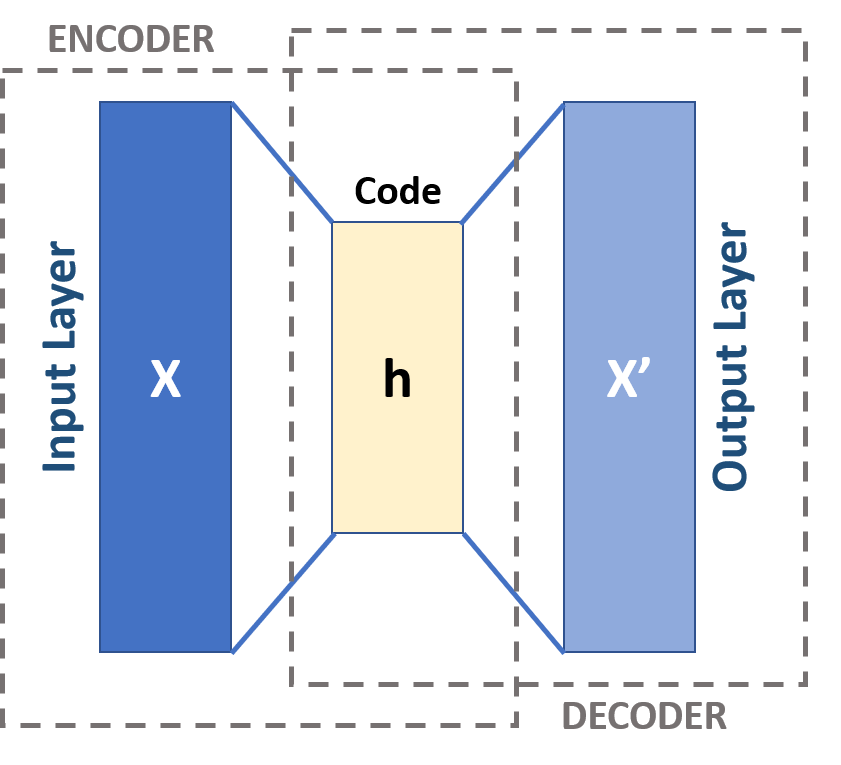## 性能对比：主成分分析 VS 自动编码器

1. PCA 只能做线性变换；而自动编码器既可以做线性变换，也可以做非线性变换。
2. 由于既有的 PCA 算法是十分成熟的，所以计算很快；而自动编码器需要通过梯度下降算法进行训练，所以需要花费更长的时间。
3. PCA 将数据投影到若干正交的方向；而自动编码器降维后数据维度并不一定是正交的。
4. PCA 是输入空间向最大变化方向的简单线性变换；而自动编码器是一种更复杂的技术，可以对相对复杂的非线性关系进行建模。
5. 依据经验来看，PCA 适用于数据量较小的场景；而自动编码器可以用于复杂的大型数据集。
6. PCA 唯一的超参数是正交向量的数量；而自动编码器的超参数则是神经网络的结构参数。
7. 单层的并且采用线性函数作为激活函数的自动编码器与 PCA 性能一致；但是多层的以非线性函数作为激活函数的自动编码器（深度自动编码器）能够具有很好的性能，虽然可能会存在过拟合，但是可以通过正则化等方式进行解决。

## 降维示例：图像数据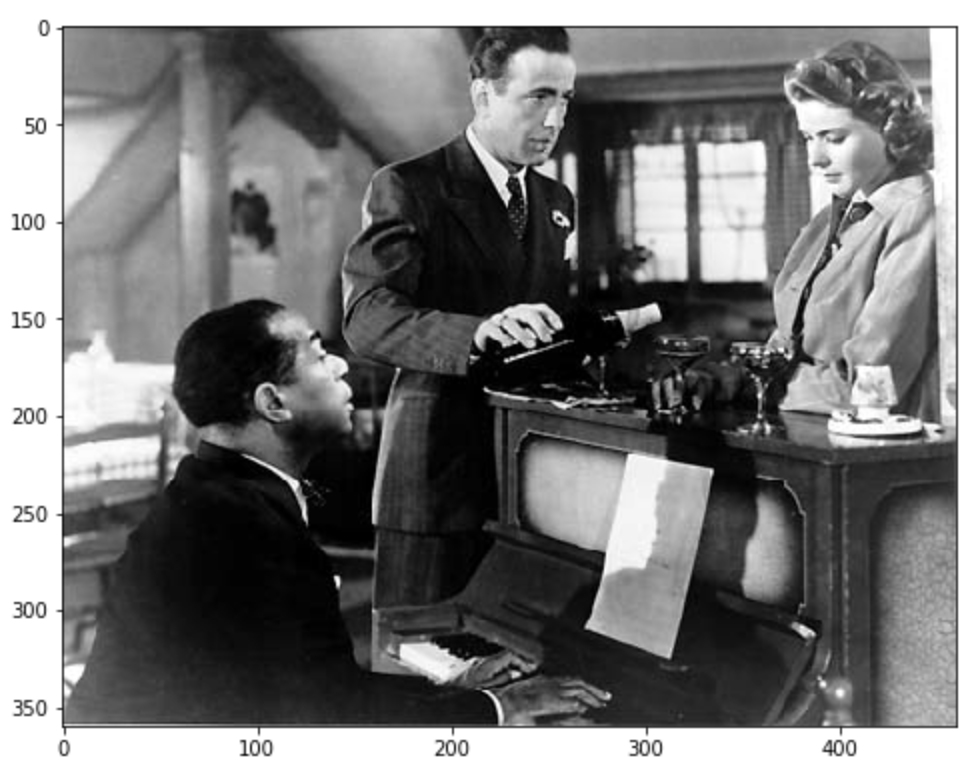### PCA 方法

``````pct_reduction = 0.10
reduced_pixel  = int( pct_reduction* original_dimensions)
#Applying PCA
pca = PCA(n_components=reduced_pixel)
pca.fit(image_matrix)
#Transforming the input matrix
X_transformed = pca.transform(image_matrix)
print("Original Input dimesnions {}".format(original_dimensions))
print("New Reduced dimensions {}".format(X_transformed.shape))
``````

``````Original Input dimesnions (360, 460)
New Reduced dimensions (360, 46)
``````

``````df_pca = pd.DataFrame(data = X_transformed,columns=list(range(X_transformed.shape)))
figure = plt.figure(figsize=(10,6))
corrMatrix = df_pca.corr()
sns.heatmap(corrMatrix, annot=False)
plt.show()
``````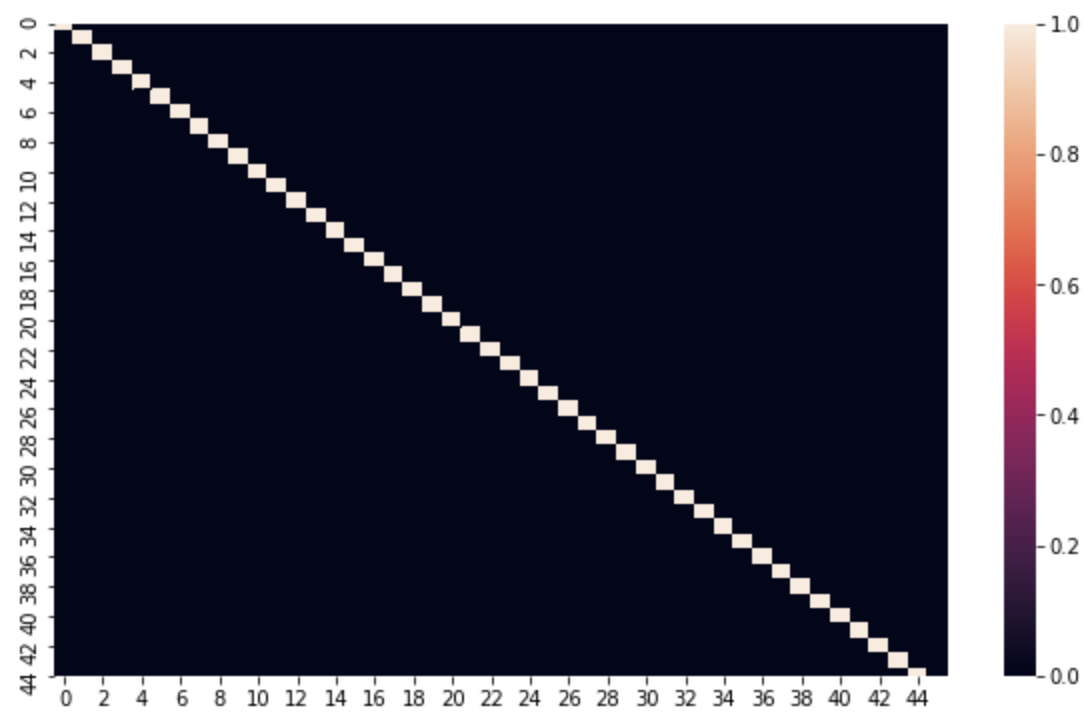PCA降维后各维度相关性

``````reconstructed_matrix = pca.inverse_transform(X_transformed)
reconstructed_image_pca = Image.fromarray(np.uint8(reconstructed_matrix))
plt.figure(figsize=(8,12))
plt.imshow(reconstructed_image_pca,cmap = plt.cm.gray)
``````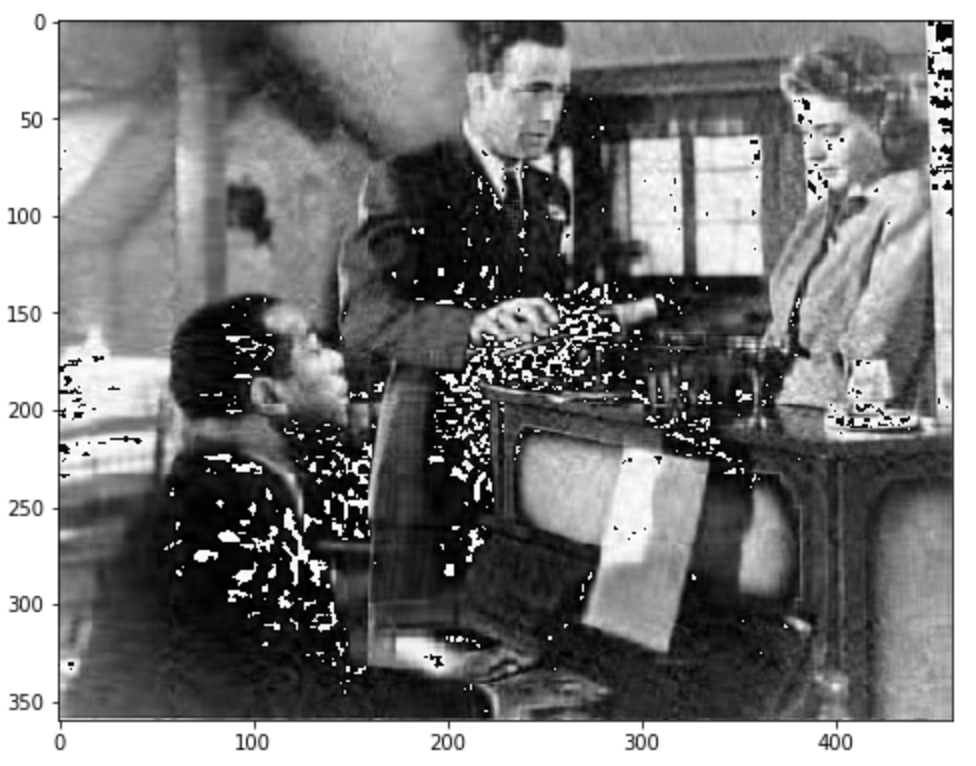PCA 图像重构

``````def my_rmse(np_arr1,np_arr2):
dim = np_arr1.shape
tot_loss = 0
for i in range(dim):
for j in range(dim):
tot_loss += math.pow((np_arr1[i,j] - np_arr2[i,j]),2)
return round(math.sqrt(tot_loss/(dim* dim*1.0)),2)
error_pca = my_rmse(image_matrix,reconstructed_matrix)
``````

### 单层的以线性函数作为激活函数的自动编码器

``````# Standarise the Data
X_org = image_matrix.copy()
sc = StandardScaler()
X = sc.fit_transform(X_org)
# this is the size of our encoded representations
encoding_dim = reduced_pixel
# this is our input placeholder
input_img = Input(shape=(img.width,))
# "encoded" is the encoded representation of the input
encoded = Dense(encoding_dim, activation='linear')(input_img)
# "decoded" is the lossy reconstruction of the input
decoded = Dense(img.width, activation=None)(encoded)
# this model maps an input to its reconstruction
autoencoder = Model(input_img, decoded)
#Encoder
encoder = Model(input_img, encoded)
# create a placeholder for an encoded (32-dimensional) input
encoded_input = Input(shape=(encoding_dim,))
# retrieve the last layer of the autoencoder model
decoder_layer = autoencoder.layers[-1]
# create the decoder model
decoder = Model(encoded_input, decoder_layer(encoded_input))
autoencoder.fit(X, X,
epochs=500,
batch_size=16,
shuffle=True)
encoded_imgs = encoder.predict(X)
decoded_imgs = decoder.predict(encoded_imgs)
``````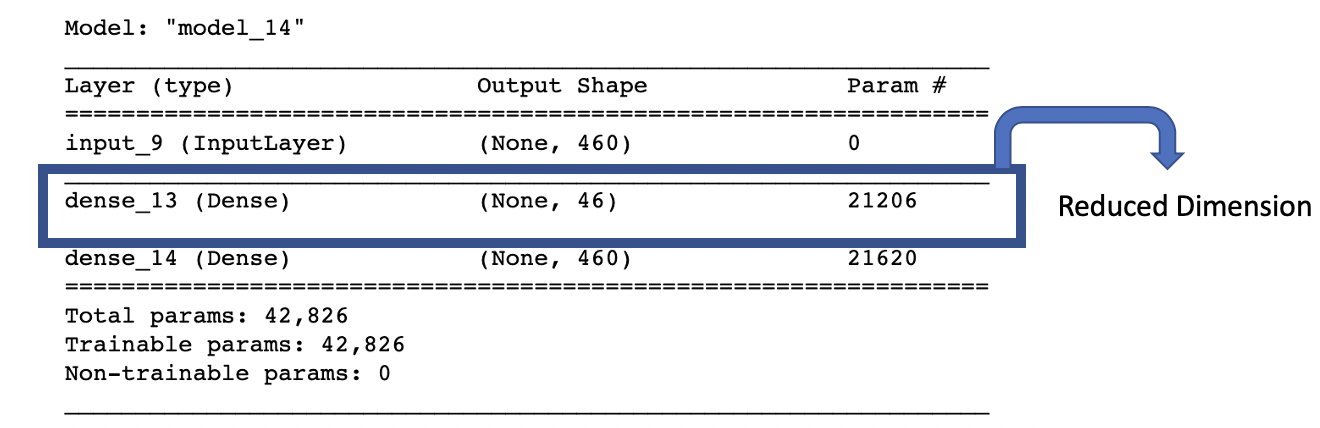``````df_ae = pd.DataFrame(data = encoded_imgs,columns=list(range(encoded_imgs.shape)))
figure = plt.figure(figsize=(10,6))
corrMatrix = df_ae.corr()
sns.heatmap(corrMatrix, annot=False)
plt.show()
``````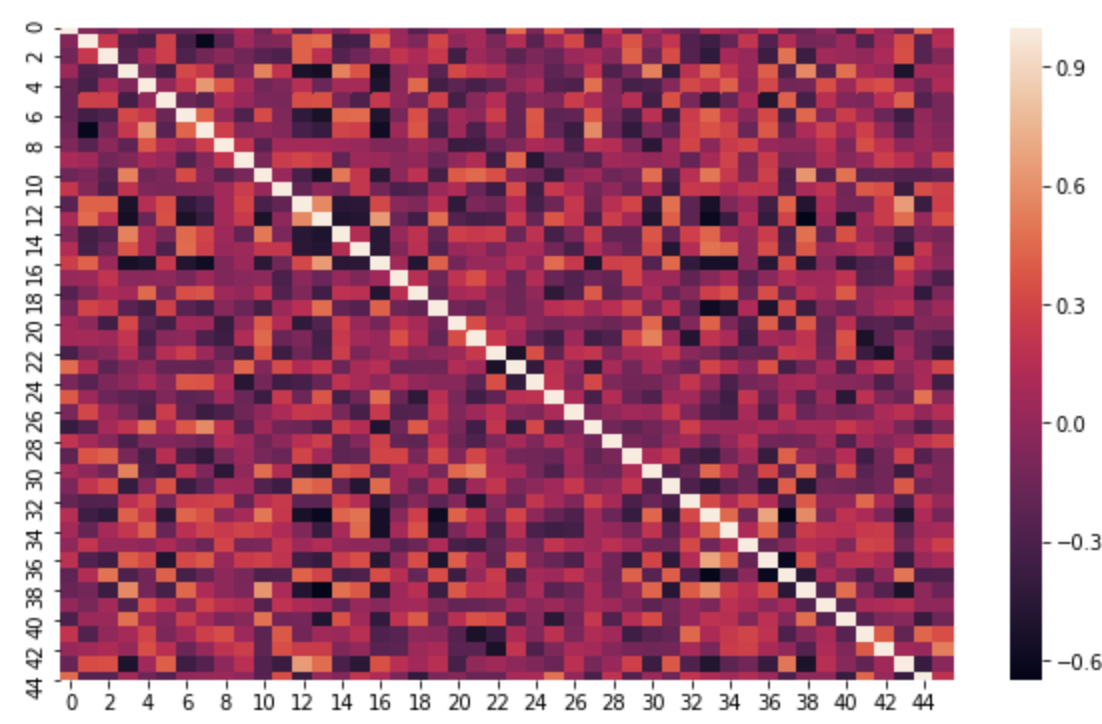``````X_decoded_ae = sc.inverse_transform(decoded_imgs)
reconstructed_image_ae = Image.fromarray(np.uint8(X_decoded_ae))
plt.figure(figsize=(8,12))
plt.imshow(reconstructed_image_ae,cmap = plt.cm.gray)
``````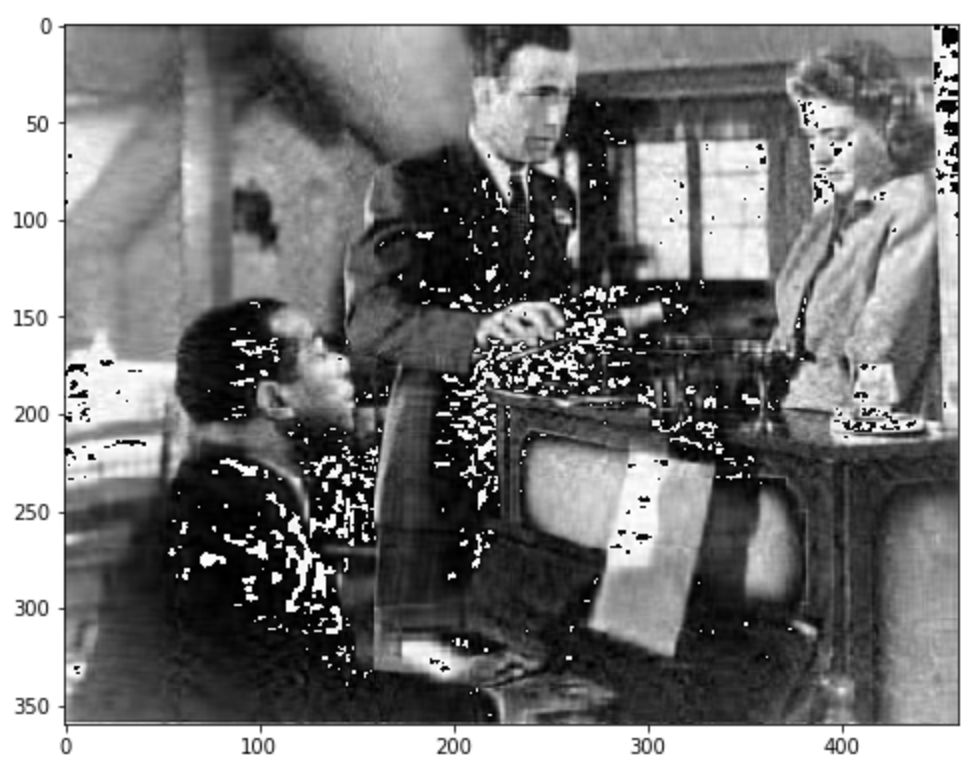``````error_ae = my_rmse(image_matrix,X_decoded_ae)
``````

### 三层的以非线性函数为激活函数的自动编码器

``````input_img = Input(shape=(img.width,))
encoded1 = Dense(128, activation='relu')(input_img)
encoded2 = Dense(reduced_pixel, activation='relu')(encoded1)
decoded1 = Dense(128, activation='relu')(encoded2)
decoded2 = Dense(img.width, activation=None)(decoded1)
autoencoder = Model(input_img, decoded2)
autoencoder.fit(X,X,
epochs=500,
batch_size=16,
shuffle=True)
# Encoder
encoder = Model(input_img, encoded2)
# Decoder
decoder = Model(input_img, decoded2)
encoded_imgs = encoder.predict(X)
decoded_imgs = decoder.predict(X)
``````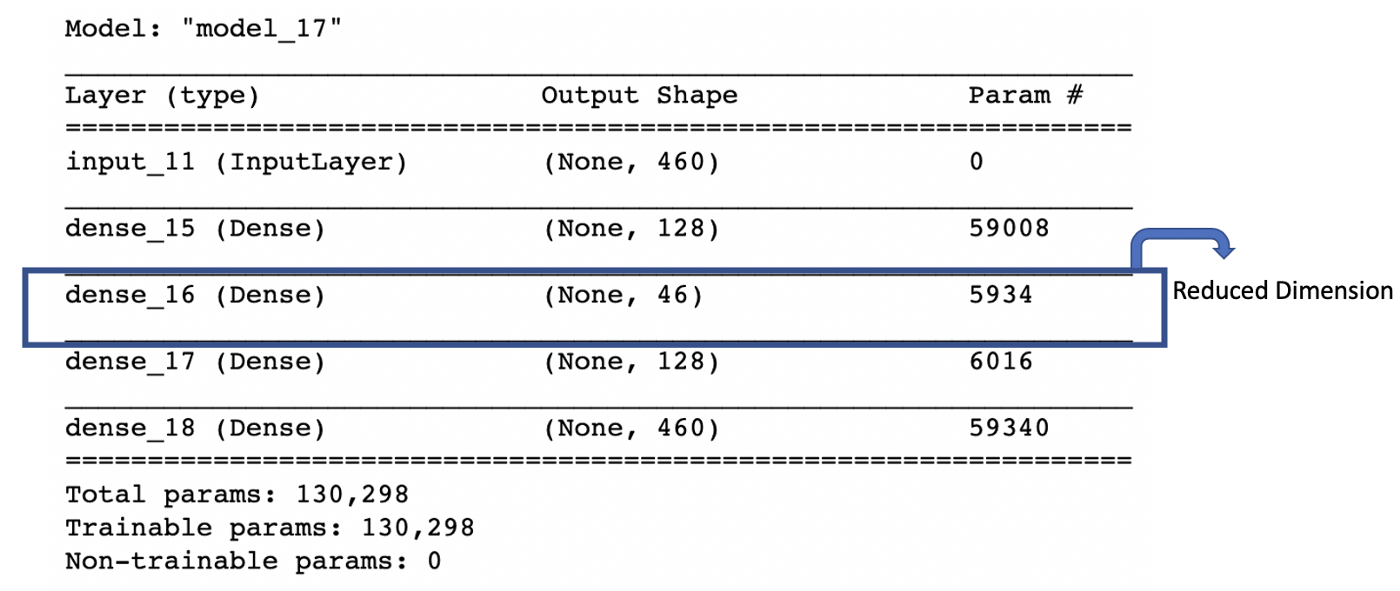``````X_decoded_deep_ae = sc.inverse_transform(decoded_imgs)
reconstructed_image_deep_ae = Image.fromarray(np.uint8(X_decoded_deep_ae))
plt.figure(figsize=(8,12))
plt.imshow(reconstructed_image_deep_ae,cmap = plt.cm.gray)
``````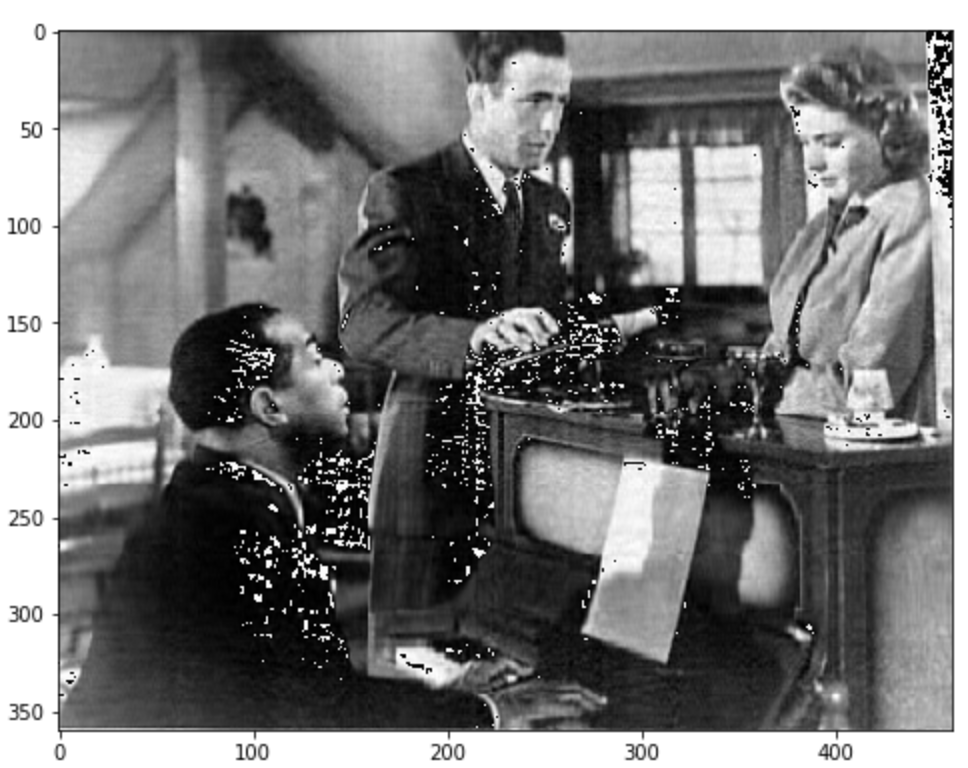``````error_dae = my_rmse(image_matrix,X_decoded_deep_ae)
``````

## 总结

deephub翻译组：Oliver Lee

### ABAP调用视图-程序员宅基地

CALL FUNCTION ‘VIEW_MAINTENANCE_CALL’EXPORTINGaction = ‘U’view_name = view_nameno_warning_for_clientindep = ‘X’EXCEPTIONSclient_reference = 1foreign_lock = 2invalid_action _abap调用视图

### chrome控制台酷炫主题_chorme 控制台主题-程序员宅基地

1.在浏览器URL栏里输入：chrome://flags/#enable-devtools-experiments，将“disabled”改为“enabled”2. command + shift + i 打开开发者工具 ,点击下图所示：打开调试工具设置setting选择experimens选项，勾选Allow custom UI themes3. 打开chrome应用商店下载自己觉得酷炫..._chorme 控制台主题

### 【Java字符串】正则表达式匹配_java 字符串正则匹配kg g-程序员宅基地

Java中经常会碰到一道题，就是字符串的匹配。正常做的话是一道难度比较大的题目，且比较复杂。其实可以通过内置的Pattern类快速解决。import java.util.regex.*;public boolean match (String str, String pattern) { return Pattern.matches(pattern, str); }..._java 字符串正则匹配kg g

### AutomationException: 0x8004033a - File creation error. in 'esri.Output' -程序员宅基地

AutomationException: 0x8004033a - File creation error. in esri.Output at com.esri.arcgis.output.ExportJPEG.finishExporting(Unknown Source) arcgis异常处理：原因就是输出图片的那个盘符的属性为共享，把它设为不共享就可以了，或者你还一个盘符或者目录试试。今_esri.output

### 掩码、子网掩码及子网划分_掩码和子网掩码的区别-程序员宅基地

1 掩码（这里的应该叫掩码，只有划分了子网才能称为子网掩码）（子网）掩码用来界定IP地址的网络标识部分和主机地址掩码是一个32位的二进制数，当用掩码和IP地址逐位做与（and）运算时，就可以得出该IP地址的网络地址下表为分类网络的子网掩码的二进制形式与十进制形式子网掩码与IP地址做与运算，计算网络地址CIDR（无类别域间路由选择）记法：202.194.36.38/242 划分子网–子网掩码_掩码和子网掩码的区别